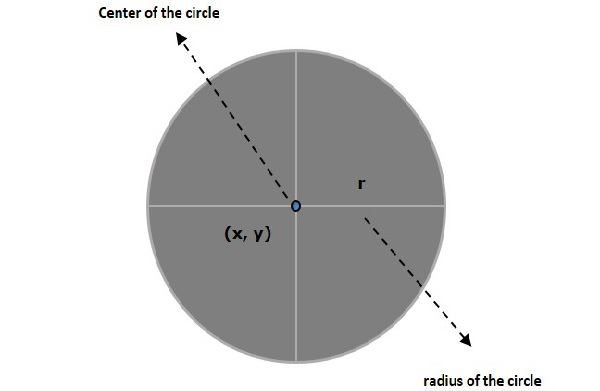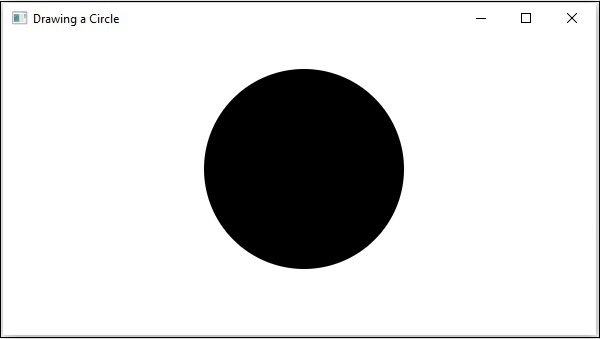# JavaFX - 2D Shapes Circle

A circle is the locus of all points at a fixed distance (radius of circle) from a fixed point (the centre of circle). In other words, a circle is a line forming a closed loop, every point on which is a fixed distance from a centre point.

A circle is defined by two parameters namely −

• Centre − It is a point inside the circle. All points on the circle are equidistant (same distance) from the centre point.

• Radius − The radius is the distance from the centre to any point on the circle. It is half the diameter.In JavaFX, a circle is represented by a class named Circle. This class belongs to the package javafx.scene.shape.

By instantiating this class, you can create a Circle node in JavaFX.

This class has 3 properties of the double datatype namely −

• centerX − The x coordinate of the center of a circle.

• centerY − The y coordinate of the center of a circle.

To draw a circle, you need to pass values to these properties, either by passing them to the constructor of this class, in the same order, at the time of instantiation, as follows −

```Circle circle = new Circle(centerx, centery, radius);
```

Or, by using their respective setter methods as follows −

```setCenterX(value);
setCenterY(value);
```

## Steps to Draw a Circle

Follow the steps given below to draw a Circle in JavaFX.

### Step 1: Creating a Class

Create a Java class and inherit the Application class of the package javafx.application and implement the start() method of this class as follows.

```public class ClassName extends Application {
@Override
public void start(Stage primaryStage) throws Exception {
}
}
```

### Step 2: Creating a Circle

You can create a circle in JavaFX by instantiating the class named Circle which belongs to a package javafx.scene.shape, instantiate this class as follows.

```//Creating a circle object
Circle circle = new Circle();
```

### Step 3: Setting Properties to the Circle

Specify the x, y coordinates of the center of the circle and the radius of the circle by setting the properties X, Y, and radius using their respective setter methods as shown in the following code block.

```circle.setCenterX(300.0f);
circle.setCenterY(135.0f);
```

### Step 4: Creating a Group Object

In the start() method, create a group object by instantiating the class named Group, which belongs to the package javafx.scene.

Pass the circle (node) object, created in the previous step, as a parameter to the constructor of the Group class, in order to add it to the group as follows −

```Group root = new Group(circle);
```

### Step 5: Creating a Scene Object

Create a Scene by instantiating the class named Scene which belongs to the package javafx.scene. To this class, pass the Group object (root), created in the previous step.

In addition to the root object, you can also pass two double parameters representing height and width of the screen along with the object of the Group class as follows.

```Scene scene = new Scene(group ,600, 300);
```

### Step 6: Setting the Title of the Stage

You can set the title to the stage using the setTitle() method of the Stage class. The primaryStage is a Stage object which is passed to the start method of the scene class, as a parameter.

Using the primaryStage object, set the title of the scene as Sample Application as follows.

```primaryStage.setTitle("Sample Application");
```

### Step 7: Adding Scene to the Stage

You can add a Scene object to the stage using the method setScene() of the class named Stage. Add the Scene object prepared in the previous steps using this method as follows.

```primaryStage.setScene(scene);
```

### Step 8: Displaying the Contents of the Stage

Display the contents of the scene using the method named show() of the Stage class as follows.

```primaryStage.show();
```

### Step 9: Launching the Application

Launch the JavaFX application by calling the static method launch() of the Application class from the main method as follows.

```public static void main(String args[]){
launch(args);
}
```

### Example

Following is a program which generates a circle using JavaFX. Save this code in a file with the name CircleExample.java.

```import javafx.application.Application;
import javafx.scene.Group;
import javafx.scene.Scene;
import javafx.stage.Stage;
import javafx.scene.shape.Circle;

public class CircleExample extends Application {
@Override
public void start(Stage stage) {
//Drawing a Circle
Circle circle = new Circle();

//Setting the properties of the circle
circle.setCenterX(300.0f);
circle.setCenterY(135.0f);

//Creating a Group object
Group root = new Group(circle);

//Creating a scene object
Scene scene = new Scene(root, 600, 300);
//Setting title to the Stage
stage.setTitle("Drawing a Circle");

stage.setScene(scene);

//Displaying the contents of the stage
stage.show();
}
public static void main(String args[]){
launch(args);
}
}
```

Compile and execute the saved java file from the command prompt using the following commands.

```javac CircleExample.java
java CircleExample
```

On executing, the above program generates a javaFx window displaying a circle as shown below.javafx_2d_shapes.htm